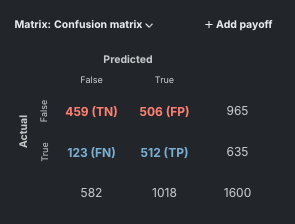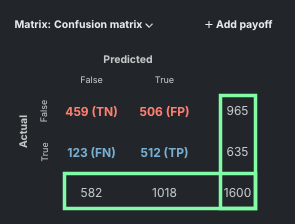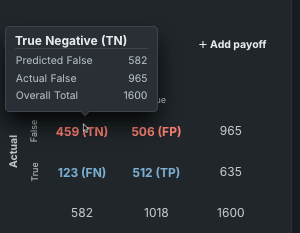Click in-app to access the full platform documentation for your version of DataRobot.

# Confusion matrix¶

The ROC Curve tab provides a confusion matrix that lets you evaluate accuracy by comparing actual versus predicted values. The confusion matrix is a table that reports true versus predicted values. The name “confusion matrix” is used because the matrix shows whether the model is confusing two classes (consistently mislabeling one class as another class).

## Evaluate accuracy using the confusion matrix¶

1. Select a model on the Leaderboard and navigate to Evaluate > ROC Curve.

2. Select a data source and set the display threshold. The confusion matrix displays on the right side of the ROC Curve tab.## Analyze the confusion matrix¶

The sample confusion matrix above is based on use case 2.

• Each column of the matrix represents the instances in a predicted class (predicted not readmitted, predicted readmitted).

• Each row represents the instances in an actual class (actually not readmitted, actually readmitted). If you look at the Actual axis on the left in the example above, True corresponds to the blue row and represents the positive class (1 or readmitted), while False corresponds to the red row and represents the negative class (0 or not readmitted).

• The matrix displays totals by row and column:• Total correct predictions are TP +TN; total incorrect predictions are FP + FN. You can interpret the sample matrix as follows (reading left to right, top to bottom) for use case 2:

Value Model prediction
True Negative (TN) 1207 patients predicted to not readmit that actually did not readmit.
False Positive (FP) 3594 patients predicted to readmit, but actually did not readmit.
False Negative (FN) 1504 patients predicted to not readmit, but actually did readmit.

Note

The Prediction Distribution graph uses these same values and definitions.

The confusion matrix facilitates more detailed analysis than relying on accuracy alone. Accuracy yields misleading results if the dataset is unbalanced (great variation in the number of samples in different classes), so it is not always a reliable metric for the real performance of a classifier.

When smart downsampling is enabled, the confusion matrix totals may differ slightly from the size of the data partitions (validation, cross-validation, and holdout). This is largely due to a rounding error. In actuality, rows from the minority class are always assigned a "weight" of 1 (not to be confused with the weight set in Advanced options and therefore never removed during downsampling. Only rows from the majority class get a "weight" greater than 1 and are potentially downsampled.

To view total counts

If you hover over a cell in the matrix (for example, the True Negative cell in the top left), you can see the total count as a numeric or percentage (total count as a numeric shown here):Updated January 20, 2023